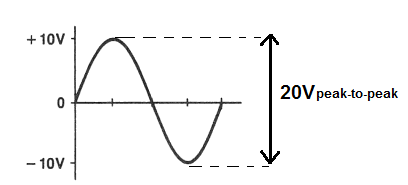﻿ What is Peak Voltage (Vp)? ﻿# What is Peak Voltage (VP)?Peak voltage, VP, is a voltage waveform which is measured from the horizontal axis (at the 0 height reference mark) to the top of the waveform, called the crest of the waveform.

Below you can see what is meant by the horizontal axis and the crest of the waveform.Again, the horizontal axis is at the 0 reference line, meaning it is 0 height above the positive voltage and 0 height below the negative voltage, meaning it is right between the positive and negative voltage waveforms. Remember, AC (alternating current) signals are signals that change polarity many times. For example, a 60Hz AC signal goes from positive to negative voltage 60 times in one second. The signal goes to its peak positive voltage, then decreases and decreases, until it hits the exact middle, which is the 0 height reference line. Then it changes polarity and reaches its peak negative voltage and then goes back down to the middle degree reference line, back to positive voltage- and this cycle repeats over and over again. This 0 height reference line is the exact middle of the signal's transition from peak positive voltage to the peak negative voltage.

This 0 height reference line gives the reference so that we can measure from it to the waveform's peak voltage, or crest. This is called the peak voltage.

Peak voltage represents the amplitude of a waveform. It shows how large the magnitude of a waveform gets from the 0 horizontal axis line to the peak of the waveform.

You can see in this example above that the peak voltage of the waveform is 10V. The top of the waveform is 10V and the horizontal axis represents 0. So 10-0=10V.

There is another type of voltage called the peak-to-peak voltage. Sometimes peak voltage and peak-to-peak voltage are confused with each other. We will show the difference between them now. While peak voltage is the voltage from the 0 horizontal reference line to the top of the waveform, the peak-to-peak voltage is the voltage of the full waveform, all the way from the peak of the negative voltage to the peak of the positive voltage. Therefore, the peak-to-peak voltage is double the value of the peak voltage waveform. The waveform above is now shown below with regard to peak voltage, not peak-to-peak.So peak voltage is exactly one half the value of the peak-to-peak voltage waveform.

### Example of Peak Voltage

You will encounter peak voltage in many environments. Many times when voltages are given, they are given in their peak voltages. However, never assume a voltage is the peak voltage unless directly specified as that. For example, the voltage should say at the end, VP. For example, 60VP. If unspecified, the voltage can be voltage RMS or the average voltage.

Let's go over a real life example of VP in an item you see regularly in buildings everywhere, including your home- an AC outlet. As you may know, an AC oulet outputs 120V. This voltage is not the peak voltage. This is the RMS voltage. If you need an explanation of RMS voltage, see RMS Voltage and Current-Explained.. This same 120Vrms is 340V in peak-to-peak voltage. So in peak voltage, it is 170 volts. So the voltage which comes out of your AC outlet (United States) is 170Vpeak. This is just a common example.

### How to Calculate Peak Voltage

Peak voltage can be calculated either from the peak-to-peak voltage, rms (root mean square) voltage, or average voltage.

The formulas to calculate the VP from either of these voltages are shown below:

### How to Calculate Peak Voltage from Peak-to-peak VoltageIf you are given the peak-to-peak voltage value, you can calculate the peak voltage using the above formula.

All you have to do to obtain the VP is to multiply the peak-to-peak voltage by 0.5.

### How to Calculate the Peak Voltage from the RMS VoltageIf you are given the RMS voltage value, you can calculate the peak voltage using the above formula.

All you must do to obtain the peak value is to multiply the RMS voltage by 1.414.

### How to Calculate the Peak Voltage from Average VoltageIf you are given the average voltage value, you can calculate the peak voltage using the above formula.

All you must do to obtain the peak value is to multiply the average voltage by π/2, which is approximately 1.57.

To use our online calculator to compute peak voltage, see Peak Voltage Calculator.

Related Resources